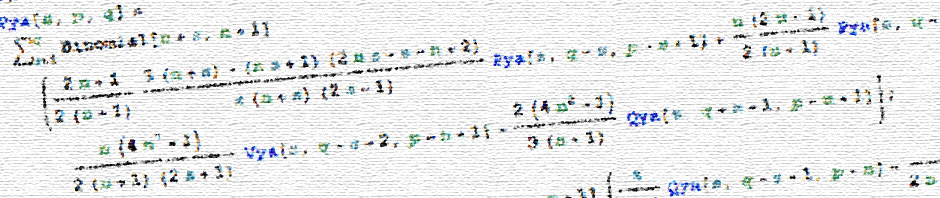# Near-field asymptotic forms of scalar resistance functionsIn my work investigating suspensions in viscoelastic fluids using Stokesian dynamics, I found that there were a considerable number of errors in the important paper

Jeffrey, D. J. & Onishi, Y., 1984 Calculation of the resistance and mobility functions for two unequal rigid spheres in low-Reynolds-number flow. Journal of Fluid Mechanics 139, 261–290.

A partial list of errata has been published by Kengo Ichiki, and some of these errors appear to have been noticed by authors using this paper in their extensions. However, I have not found a comprehensive description of how to fully generate, from scratch, the near-field asymptotic forms of scalar resistance functions,

$$X^A_{11}, X^A_{12}, Y^A_{11}, Y^A_{12}, Y^B_{11}, Y^B_{12}, X^C_{11}, X^C_{12}, Y^C_{11}, Y^C_{12},$$

$$X^G_{11}, X^G_{12}, Y^G_{11}, Y^G_{12}, Y^H_{11}, Y^H_{12}, X^M_{11}, X^M_{12}, Y^M_{11}, Y^M_{12}, Z^M_{11}, Z^M_{12}.$$

The linked-to document below attempts to rectify this.

In this, I use two later, useful papers:

Jeffrey, D. J. 1992 The calculation of the low Reynolds number resistance functions for two unequal spheres. Physics of Fluids A: Fluid Dynamics (1989-1993) 4 (1), 16–29.

Ichiki, K., Kobryn, A. E. & Kovalenko, A. 2013 Resistance functions for two unequal
spheres in linear flow at low Reynolds number with the Navier slip boundary condition. arXiv:1302.0461 [cond-mat, physics:physics]

Equations in these papers will be referenced thus:

 Jeffrey & Onishi (1984) (JO 1.1) Jeffrey (1992) (J 1) Ichiki et al. (2013) (I 1)

The full expressions can be downloaded below as a PDF from the arXiv:

• Townsend, A. K. 2018 Generating, from scratch, the near-field asymptotic forms of scalar resistance functions for two unequal rigid spheres in low-Reynolds-number flow (Corrections to Jeffrey & Onishi (1984)). arXiv:1802.08226 [physics.flu-dyn]